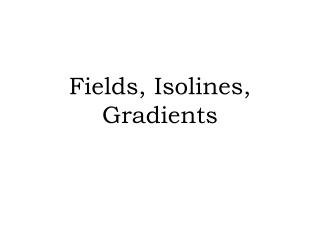DownloadDownload PresentationTélécharger la présentation- - - - - - - - - - - - - - - - - - - - - - - - - - - E N D - - - - - - - - - - - - - - - - - - - - - - - - - - -
##### Presentation Transcript

2. Fields • What is a field? • A field is any region of space that has a measurable value at every point. (Ex Temperature)

3. Isolines • What is an Isoline? • Isolines literally mean same (iso-) lines. (isosceles triangle) • They are lines that connect points of equal value in a given field. • Similar to Contour lines.

4. Common examples of isolines are: • Isotherms – Same temperature

5. Common examples of isolines are: Isobars – Same (Bar) atmospheric pressure

6. Common examples of isolines are: Contour lines – Same elevation

7. Gradients • What is Gradient? • Gradient is a rate of change (aka slope) • How do we find Gradient? • Calculated by gradient = change in field value distance • What does it mean when the lines are close together? • Spacing of isolines – the closer together the higher the gradient

8. How heat travels • How does heat travel? • Heat travels from Hot to Cold. • More speed to less speed • What is something that adds heat called? • Heat source adds heat. • What is an area where heat leaves called? • Heat sink is where heat leaves.

9. Heat Lab Conclusion • What does a thermometer measure? • Heat is a measure of? • How does heat travel horizontally? • What 2 things create heat differences? • Heat Source? (3 examples) • Heat Sink? (3 examples) • Why does hot air rise? (explain)